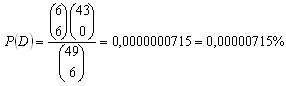sk|cz|

# Probability

1.Define and characterize probability.

Solution:

a) Standard probability definition

Let a random event meet following conditions:
• number of the events is finite
• all events have the same chance to occur
• no two events can occur in the same time

Probability of an event A equals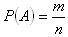, n = # of all possible events, m = number of cases favorable for the event A

Stands: 0 ≤ P(A) ≤ 1
Probability of an impossible event : P(A) = 0
Probability of a sure event: P(A) = 1

b) Conditional probability of some event A, given the occurrence of some other event B: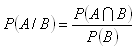c) Probability of two independent events:

P(A∩B) = P(A)P(B)

d) Probability of two mutually exclusive events:

P(AUB) = P(A) + P(B)

e) Binomial probability expression:

Let an event A occur with probability P. The probability of k occurrances of the event A in n tries equals: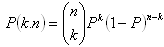f) Hypergeometric probability expression:

Let V of N elements have a property p; derived from that, N-V elements do not have the property p.
Probability of k of n randomly selected elements having the property p equals::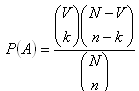2.There are 18 tickets marked with numbers 1 to 18. What‘s the probability of selecting a ticket having the following property:

a) even number
b) number divisible by 3
c) prime number
d) number divisible by 6
Solution: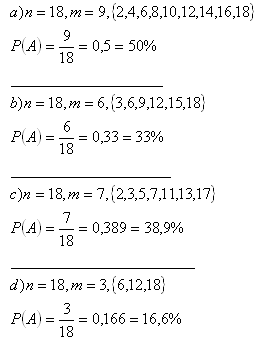3.Determine the probability of following results when throwing 2 playing cubes (a red one and a blue one):

a) sum equals to 8
b) sum divisible by 5
c) even sum
Solution: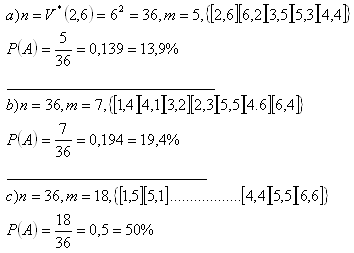4.A gambler playing with 3 playing cubes wants to know weather to bet on sum 11 or 12. Which of the sums will occur more probably?

Solution: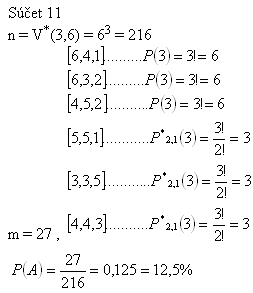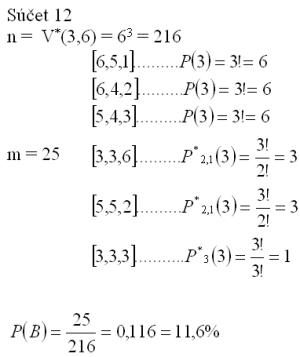The gambler should bet on 11, as P(11) > P(12).

5.82 170 of 100 000 children live 40 years and 37 930 of 100 000 children live 70 years. Determine the probability of a 40 years old person to live 70 years.

Solution:

(Conditional probability)

A – live 70 years, P(A) = 0,3793
B – live 40 years, P(B) = 0,8217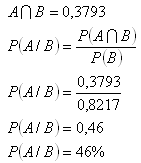The probability equals 46%.

6.In a town there are 4 crossroads with trafic lights. Each trafic light opens or closes the traffic with the same probability of 0.5. Determine the probability of:

a) a car crossing the first crossroad without stopping
b) a car crossing first two crossroads without stopping
c) a car crossing all the crossroads (4) without stopping
Solution: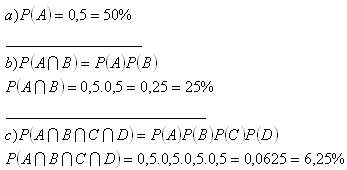7.32 playing cards include 4 aces and 12 figures. Determine the probability of a randomly selected card to be an ace or a figure.

Solution:

Probability of two mutually exclusive events

A – selected ace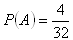B - selected figure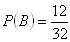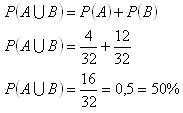The probability of selecting an ace or a figure equals 50%.

8.Determine the probability of 3 of 5 born children being sons if the probability of a children to be a boy equals P(A) = 0,51.

Solution:

Binomial probability expression.
n = 5, k = 3, P = 0,51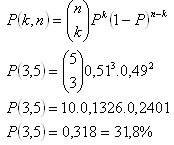The probability equals 31,8 %.

9. There are 16 cola bottles on the table. 10 of them are filled by Coca Cola and 6 of them are filled by Pepsi. Determine the probability of 4 randomly selected bottles to include 2 Coca Cola and 2 Pepsi bottles.

Solution:

Hypogeometric probability expression.

N = 16 (# all bottles)
V = 10 (# Coca Cola)
N-V = 6 (# Pepsi)
n = 4 (# randomly selected bottles)
k = 2 (# selected Coca Cola)
n – k = 2 (# selected Pepsi)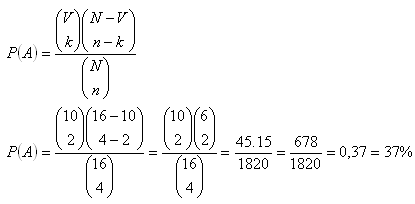The probability equals P(A) = 37 %.

10.In a game of chance 6 of 49 numbers are the winning numbers. Determine the probability of reaching:

a) matching 3 of 6
b) matching 4 of 6
c) matching 5 of 6
d) matching 6 of 6
Solution:

a) Matching 3 of 6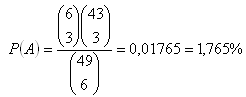b) Matching 4 of 6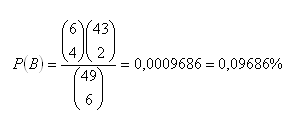c) Matching 5 of 6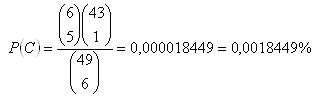d) Matching 6 of 6